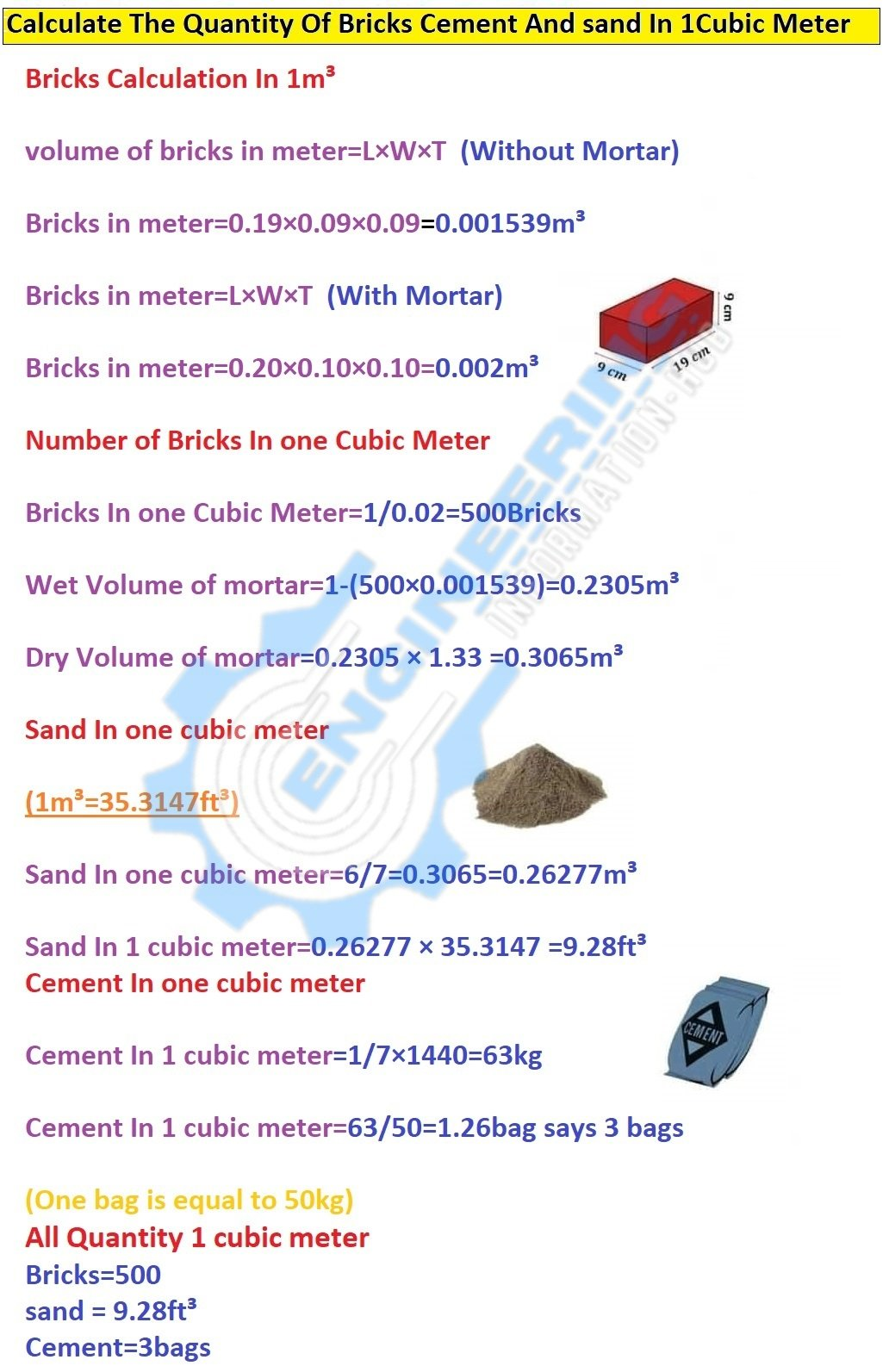Civil Engineering

# Calculate The Quantity Of The Bricks Cement And sand In One Cubic Meter

## Calculate The Quantity Of Bricks in 1m3| Calculate The Quantity Of Cement in 1m3|Calculate The Quantity Of Sand in 1m3

### Calculate The Quantity Of TheBricks Cement And sandIn One Cubic Meter

In this article, I will calculate the quantity of bricks sand, and cement in one cubic meter. we have some given data about the ratio of mortar. with the help of this, we can calculate the exact quantity of cement sand and Bariks. Calculate The Quantity Of The Bricks Cement And sand In One Cubic Meter. Calculate The Number Of Bricks in 1m3| Calculate The Quantity Of Cement in 1m3|Calculate The Quantity Of Sand in 1m3

### Given Data

The ratio of mortar= 6:1

sum of ratio=6+1=7

Mortar Thicknes=10mm=0.01m

### Solution

We Know

The Density of cement =1440kg/m³

### Bricks Calculation In 1m³

As per Par Standard The volume of bricks in meter=L×W×T  (Without Mortar)

The volume of bricks in meter=0.19×0.09×0.09=0.001539m³

The volume of bricks in meter=L×W×T  (With Mortar)

The volume of bricks in meter=0.20×0.10×0.10=0.002m³

Now We Calculate the Number of Bricks In one Cubic Meter

### Number of Bricks In one Cubic Meter

Number of Bricks In one Cubic Meter=1m³/volume of  bricks with mortar

Number of Bricks In one Cubic Meter=1/0.02=500Bricks

Wet Volume of mortar=1m³-(total bricks×volume of bricks with mortar)

Wet Volume of mortar=1-(500×0.001539)=0.2305m³

Dry Volume of mortar=wet Volume of mortar × constant value

Dry Volume of mortar=0.2305 × 1.33 =0.3065m³

### Sand In one cubic meter

Sand In one cubic meter=ratio of sand/ sum of the ratio ×Dry volume of mortar

Sand In one cubic meter=6/7=0.3065=0.26277m³

Sand In one cubic meter=0.26277 × 35.3147 =9.28ft³   (1m³=35.3147ft³)

### Cement In one cubic meter

Cement In one cubic meter=ratio of cement/ sum of the ratio × density of cement

Cement In one cubic meter=1/7×1440=63kg

Cement In one cubic meter=63/50=1.26bag says 3 bags                      (One bag is equal to 50kg)

4. ### How To Calculate The Bricks Quantity For The Room